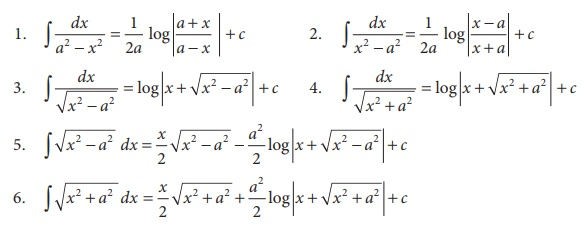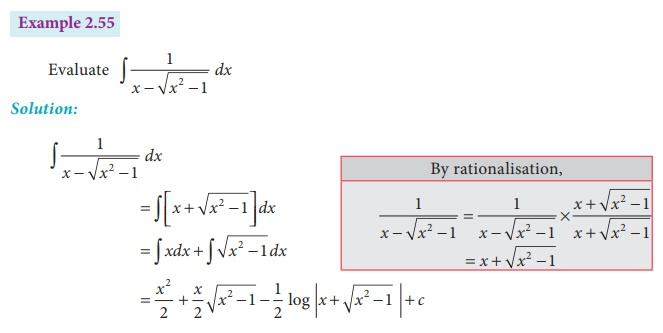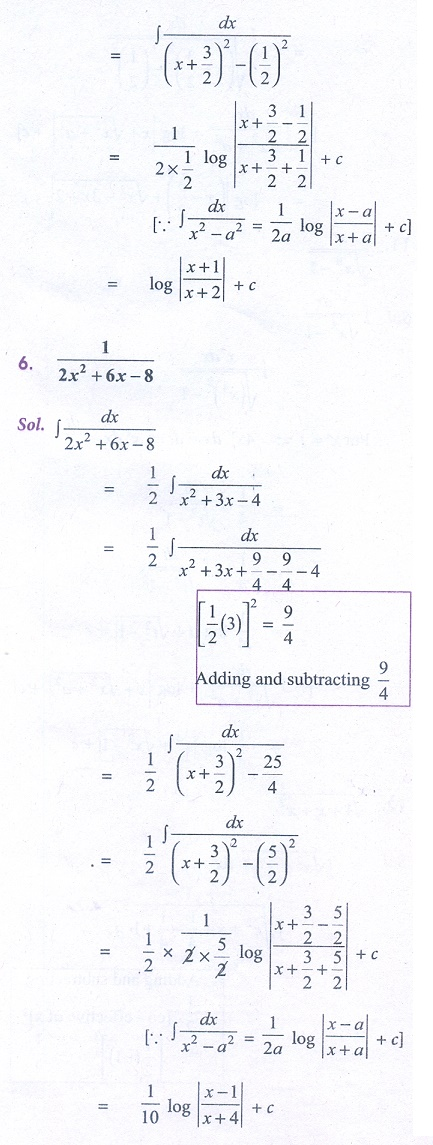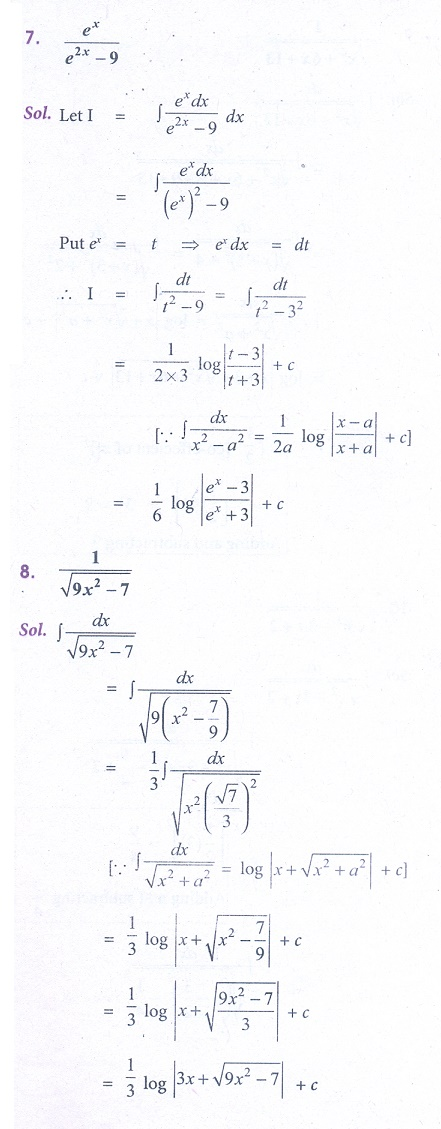Home | | Business Maths 12th Std | Some special types of Integrals Method

# Some special types of Integrals Method

Maths: Integral Calculus: Indefinite Integrals: Methods of integration: Some special types of Integrals: Type VII : Exercise and Example Solved Problems with Answer, Solution

Some special types of Integrals

Type: VII

To evaluate the integrals of the formandfirst we have to express ax2 + bx + c as the sum or difference of two square terms [completing the squares], that is ( x + α )2 + β2 (orx + α )2 − β2 (or2 − ( x + α)2 and apply the suitable formula from the formulae given below.ExampleExercise 2.7

Integrate the following with respect to x